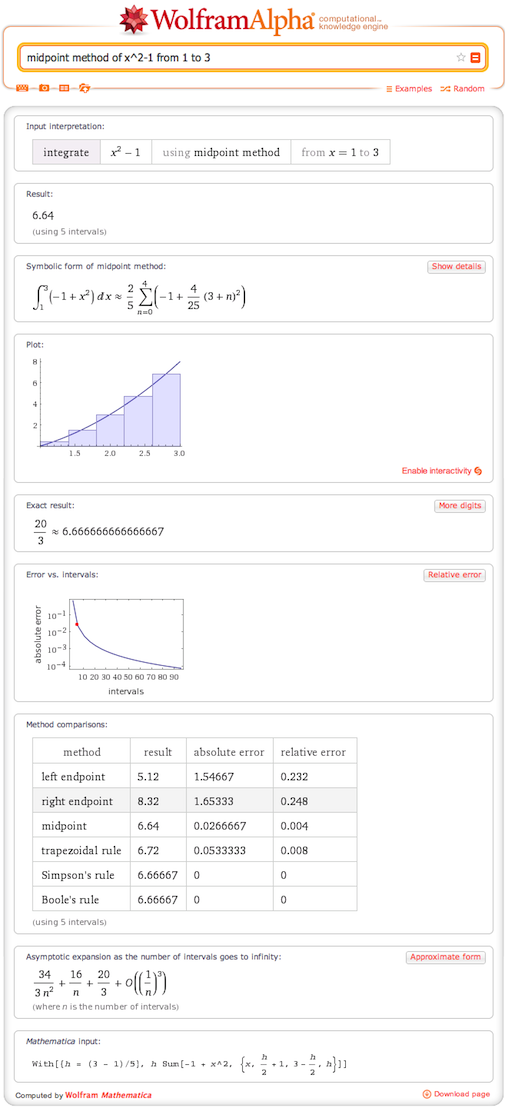# Numerical Methods: Integration

July 11, 2013 —Comments Off

As we continue to expand the functionality of Wolfram|Alpha, we want to include not only the symbolic and exact results, but also allow you the option to explore the numerical approximations for solving mathematical problems such as differential equations and integrals. These methods, both simple and complex, continue to underpin many of our modern day calculations.

Integration is where many of us have encountered numerical approximations to mathematical problems. These simple approximations are used in high school and introductory college classes to form a starting point for more advanced methods of solving integrals.

One of the most basic methods for approximating an integral is the Riemann sum. In this method you divide the region you are integrating over into a number of intervals. You can then find the value of the function you are integrating, called the integrand, at some point within each interval. By multiplying those values by the width of each interval and then summing, you can get an approximation to the value of the integral. A basic example of this is the midpoint method (midpoint method of x^2-1 from 1 to 3) where you calculate the value at the center of each interval.We provide the symbolic code for these calculations so that you can work out each step. Additionally we show how increasing the number of intervals affects the error, or divergence from the exact result, moving closer and closer to zero. You can also examine the asymptotic expansion to see how the result converges on the exact solution.

Faster convergence can be reached with more advanced methods. Wolfram|Alpha shows the results for some of these methods so you can compare their accuracy. The two endpoint methods are simply variations on the midpoint method, adjusting where they take their value. A more accurate method is the trapezoidal method (trapezoidal method of x cos x), which averages the endpoints to calculate a value at the center of the interval.

Yet more advanced is Simpson’s rule (Simpson’s method for e^x sin x + x^2 from 0 to 4), one of the two Newton–Cotes methods currently supported. Here the calculations become more complicated, but for those interested, we provide both the general equations and how they look with your specific problem.These numerical methods can be used to obtain approximations for integrals for which there exists no closed-form solution, such as Bessel J(2,x)/(1 + x^2). By using Simpson’s rule (using Simpson’s rule integrate Bessel J(2,x)/(1 + x^2) from 1 to 3 with 10 intervals), however, we can get a result.

In addition to specifying the integration range (left endpoint method of x^5-2 x from -3 to 3), you can give the number of intervals (Boole’s rule for sin x cos x e^x from 1 to 3 in 4 intervals) or the width of the intervals (integrate x log x from 0 to 2 using right endpoint method with an interval width of 0.5). Those of you using Wolfram|Alpha Pro can utilize the CDF content to dynamically alter the number of intervals used, and in some cases, the exact method.

We hope you enjoyed this illustration of the basic numerical methods of integration and use this and other Step-by-Step functionality in Wolfram|Alpha.